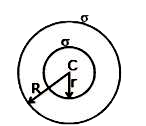Question

Updated on:30/05/2023

# A charge Q is distributed over two concentric hollow spheres of radii r and R(>r) such that the surface charge densities are equal. Find the potential at the common centre.

Text Solution

A
14πε0(2R+r)(R2+r2)Q
B
14πε0(R+r)(R2+r2)Q
C
14πε0(R+r)2(R2+r2)Q
D
14πε0(R+2r)Q2(R2+r2)
SolutionQ1=σ.4πr2
Q2=σ.4πR2
Q=4πσ(R2+r2)
σ=Q4π(R2+r2)
ve=KQr+KQ2R
==Kσ4πr2r=Kσ4πR2R=Kσ4π(r+R)
4πKQ4π(R2+r2)(r+Q)=KQ(r+Q)(R2+r2)

Transcript

hello everyone I charge capital Q is distributed over two concentric hollow sphere of radius R and capital are such that the surface charge densities are equal and you are to find out the potential at the common Centre so the radius of the two square is smaller and capital r and the total charges Q if you see here this R1 is smaller and this r 2 is equals to Capital or and these are the two squares and the charges on the two squares less the force on this is Q1 and this is Kyoto OK it is said that charge density is on the both is retained its if I write the charge density on the inner sphere 21 so it will be for a smaller square charge per unit area and for the outer sphere it will be for
capital Square from here on this post I will get cancelled out and you will get you one by two 2 is equals to R square by Oscar where is simple now that you're oneplus Q2 is given in the question is equals to you so I will write this one + Q 2 is equals to Kyon ok sunao we want to the common at the potential at the common Centre so potential at the common Centre will request to ke 21 by small Plus ke Chhotu bike app Talaq now you could put the value of Q1 and Q2 after solving these two equations this is the equation 1 and this is the equation to write ok if you seek you 1 is equals to 1 equals to 2 into our square b square so I will put you to INR to ask
I ask this is the Q1 and this is Q2 and is just too cute it's so I will write the Q 2 is equals to cure upon 1 + R square b square it ok so we got the value of cute to and now you can also calculate the value of Q one since the to 1 by 2 is equal to squares of you when will be this times of cute o r square B Square X is equals to a square by R squared value of a square b square so I will write Q R square by R square so know if you will solve this yes we will solve this now you can take a square b square common here
and you will write R square by R square into 1 + this into this will be cancelled out ok and we got the Q2 now you can just put the value of Q1 and Q2 so if I take ke common so Q1 by are so this is Q1 and if it take to 1 by our sweet will be close to you upon our times of 1 + cos square b square ok now If he's in the queue to Bhai chapter 8 so cute to buy capital are so cute to is this and ok so this will be the now you could solve it for calculation of the potential
for this is the expression for the potential at the common Centre it's over the refusal solve it you will get the potential at the centre solve it for potential at the centre so if you send this small and capital inside then you will get so I will write your ke is the common and q is also common if you see you is common here and in denominator I will write a square + Y square and if you see in denominator there will be smaller a square and it will be cancelled out by this smaller into this quality were taken outside Plus one upon again R square + Y square by our
if you will solve this you will get cake is upon our square + Y square + R R square + R square now you could see if you take a square + Y square common then you will get kq bi R squared plus R square into smaller the scatter this will be the potential at the common centre and this was asking this question so this is our answer sunao if you show the correct option you can you could choose your violetta calculated ke you are + R R squared Plus ok so you could see here ke is 1 upon 4 Pi Epsilon to the correct answer
will be thank you